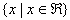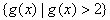Example 1

Find the domain and range of the function: g(x) = 3.2x+1 + 2.

Step 1. Find the domain.

Since the function is not representing a situation, the x can be any real number.

Therefore, the domain is.

Step 2. Find the range.

This graph is shifted up 2 and has an asymptote at the line y = 2. It also has a positive a-value.

Therefore, the range of the function is.